# 9 times tables trick: How to remember the table

Learning the multiplication tables by the students is not an easy task in itself. Our 9 times tables trick will help you to memorize. Why we discuss the time table of 9? It is even more complicated because for most children that counting nine in nine costs. Some are not good and there are others who take a long time to know the result until they learn it from memory. However, to remember the table of 9, there are a very fast tricks that is sure to please all children. This trick uses the fingers of the hands to know the result of the multiplications of the table of 9.

## 9 times tables trick

First, we have to tell the child to open their hands with all fingers extended and with the palms of the hand putting down. We have to remind the child that the little finger of the left hand represents 1, the ring finger of the same hand would be 2, the middle of the same hand would be 3 and so on until reaching the little finger of the right hand that would be equivalent to 10.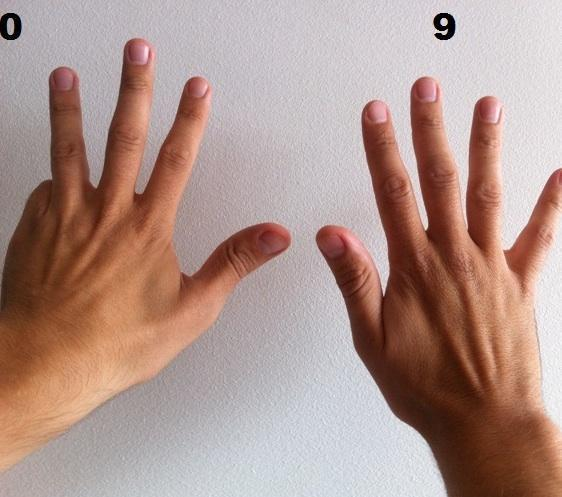### Step 9×1

To remember the multiplication table of 9, before any question in the table. For example, 9×1, this method is to take into account the number that is multiplied by 9. In this case 1, and we have to tell the child to double the finger nº 1 (little finger of the left hand). Then the result of the multiplication will always be the number of fingers that remain to the left of the bent finger, there are 0 fingers to the left, followed by the number of fingers that remain to the right of the bent finger, 9 fingers remain to the right, that is, the result would be 09.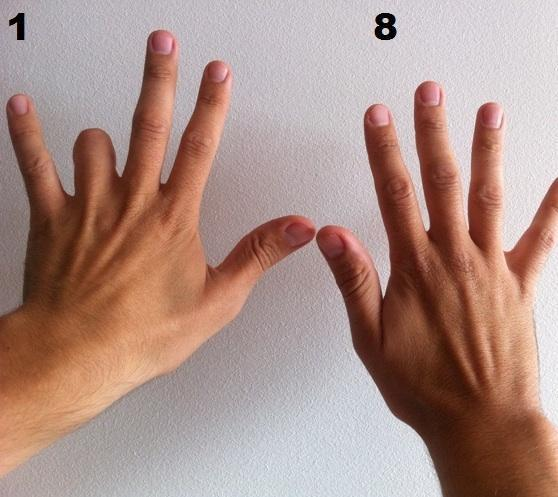### Step 9×2

At the 9×2 question, we have to tell the child to bend finger #2 (ring finger of the left hand). Then the result of the multiplication will always be the number of fingers that remain to the left of the bent finger, there is 1 finger to the left, followed by the number of fingers that remain to the right of the bent finger, there are 8 fingers to the right, that is, the result is 18.### Step 9×3

At the 9×3 question, we have to tell the child to bend finger #3 (middle finger of the left hand). Then the result of the multiplication will always be the number of fingers that remain to the left of the bent finger, 2 fingers remain to the left, followed by the number of fingers that remain to the right of the bent finger, 7 fingers remain to the right, that is, the result is 27.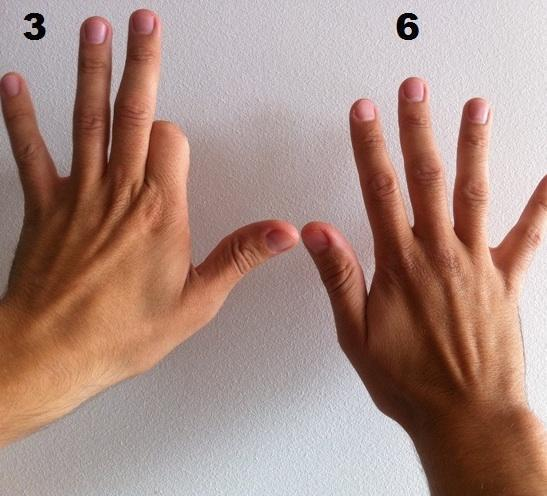### Step 9×4

To the 9×4 question, we have to tell the child to bend finger number 4 (index finger of the left hand). Then the result of the multiplication will always be the number of fingers that remain to the left of the bent finger, 3 fingers remain to the left, followed by the number of fingers remaining to the right of the bent finger, 6 fingers remain to the right, that is, the result is 36.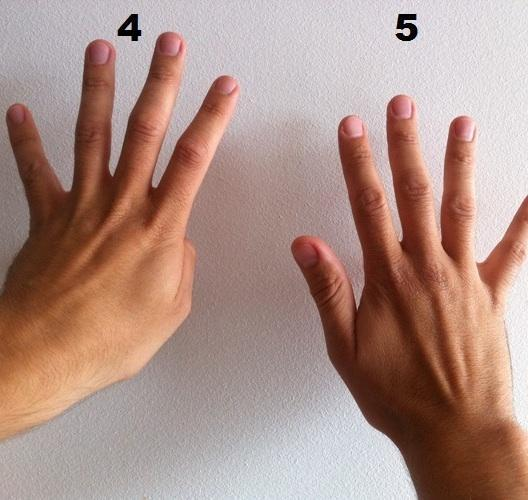### Step 9×5

At the 9×5 question, we have to tell the child to bend finger number 5 (thumb of the left hand). Then the result of the multiplication will always be the number of fingers that remain to the left of the bent finger, 4 fingers remain to the left, followed by the number of fingers that remain to the right of the bent finger, 5 fingers remain to the right, that is, the result is 45.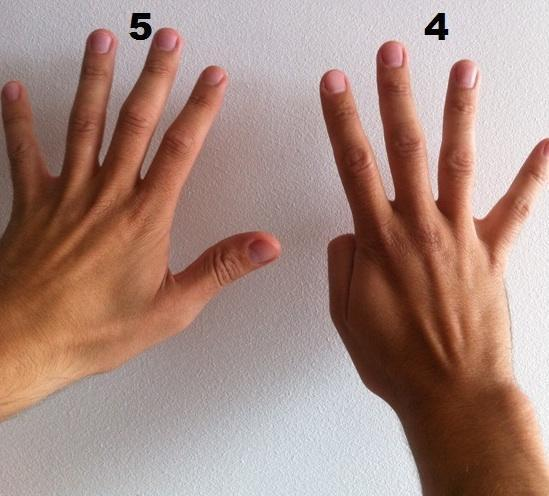### Step 9×6

To the question of 9×6, we have to tell the child to bend finger number 6 (thumb of the right hand). Then the result of the multiplication will always be the number of fingers that remain to the left of the bent finger, 5 fingers remain to the left, followed by the number of fingers that remain to the right of the bent finger, 4 fingers remain to the right, that is, the result is 54.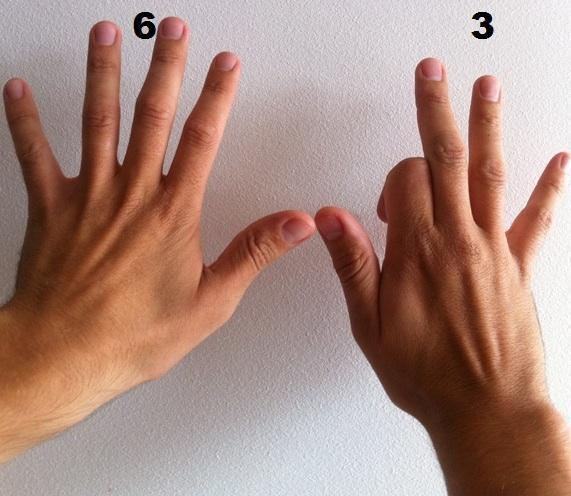### Step 9×7

To the question of 9×7, we have to tell the child to bend finger # 7 (index finger of the right hand). Then the result of the multiplication will always be the number of fingers that remain to the left of the bent finger, there are 6 fingers to the left, followed by the number of fingers that remain to the right of the bent finger, 3 fingers remain to the right, that is, the result is 63.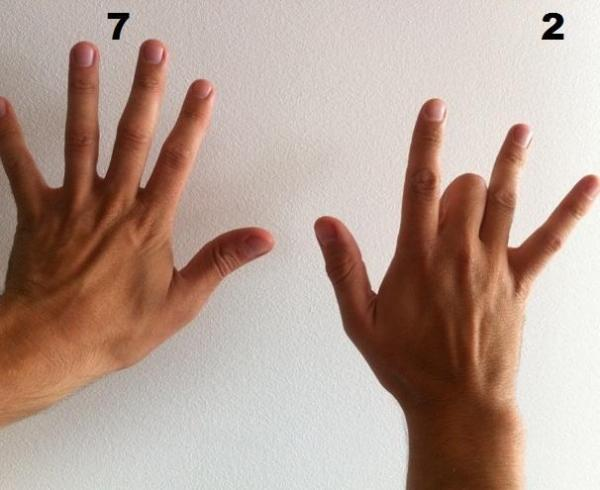### Step 9×8

To the question of 9×8, we have to tell the child to bend finger # 8 (middle finger of the right hand). Then the result of the multiplication will always be the number of fingers that remain to the left of the bent finger, 7 fingers remain to the left, followed by the number of fingers that remain to the right of the bent finger, 2 fingers remain to the right, that is, the result is 72.### Step 9×9

To the 9×9 question, we have to tell the child to bend the 9th finger (ring finger of the right hand). Then the result of the multiplication will always be the number of fingers that remain to the left of the bent finger. There are 8 fingers to the left, followed by the number of fingers that remain to the right of the bent finger, there is 1 finger to the right, that is, the result is 81.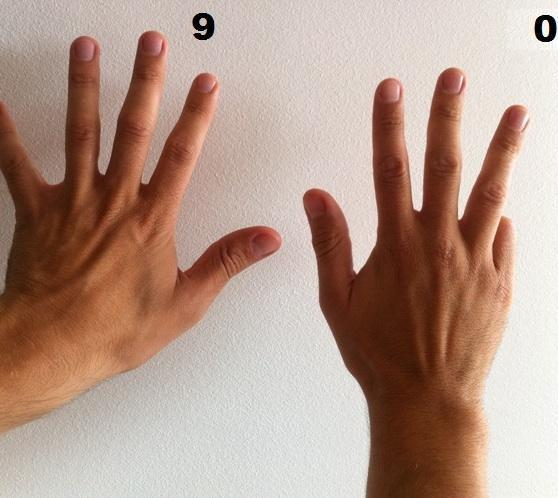### Final step

At the question of 9×10, we have to tell the child to bend finger # 10 (little finger of the right hand). Then the result of the multiplication will always be the number of fingers that remain to the left of the bent finger, there are 9 fingers to the left, followed by the number of fingers that remain to the right of the bent finger, there are 0 fingers to the right, that is, the result is 90.

If you think that the 9 times tables trick is helpful, don’t forget to share.